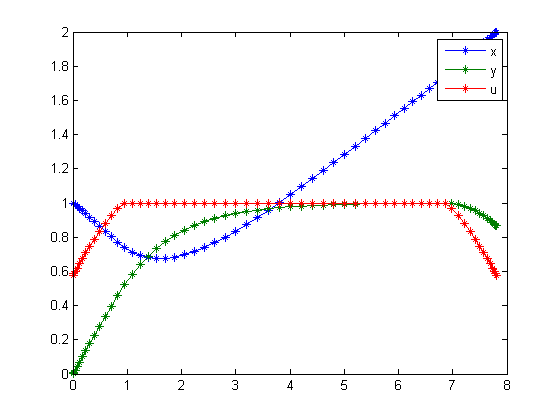30  Dielectrophoresis Particle Control

Time-Optimal Control of a Particle in a Dielectrophoretic System, Dong Eui Chang, Nicolas Petit, and Pierre Rouchon

30.1  Problem Description

Find u over t in [0; t_F ] to minimize:

 J = tf

subject to:

 dx dt
= y*u+alpha*u2
 dy dt
= −c*y+u
 |u| <= 1
alpha = −
 3 4

 c = 1
 [x0  y0] = [1  0]
 xtf = 2

Reference: 

30.2  Problem setup

toms t
toms t_f
p = tomPhase('p', t, 0, t_f, 60);
setPhase(p);

tomStates x y
tomControls u

% Initial guess
x0 = {t_f == 10
icollocate({
x == 1+1*t/t_f
y == t/t_f
})
collocate(u == 1)};

% Box constraints
cbox = {
sqrt(eps) <= icollocate(x)
sqrt(eps) <= collocate(y)
1         <= t_f <= 100
-1        <= collocate(u) <= 1};

% Boundary constraints
cbnd = {initial({x == 1; y == 0})
final({x == 2})};

% ODEs and path constraints
ceq = collocate({
dot(x) == y.*u-3/4*u.^2
dot(y) == -y+u});

% Objective
objective = t_f;

30.3  Solve the problem

options = struct;
options.name = 'Dielectrophoresis Control';
solution = ezsolve(objective, {cbox, cbnd, ceq}, x0, options);
t = subs(collocate(t),solution);
x = subs(collocate(x),solution);
y = subs(collocate(y),solution);
u = subs(collocate(u),solution);
Problem type appears to be: lpcon
Starting numeric solver
===== * * * =================================================================== * * *
TOMLAB - Tomlab Optimization Inc. Development license  999001. Valid to 2011-02-05
=====================================================================================
Problem: ---  1: Dielectrophoresis Control      f_k       7.811292811901784800
sum(|constr|)      0.000001365448751008
f(x_k) + sum(|constr|)      7.811294177350536200
f(x_0)     10.000000000000000000

Solver: snopt.  EXIT=0.  INFORM=1.
SNOPT 7.2-5 NLP code
Optimality conditions satisfied

FuncEv    1 ConstrEv   26 ConJacEv   26 Iter   25 MinorIter  218
CPU time: 0.281250 sec. Elapsed time: 0.281000 sec.

30.4  Plot result

figure(1);
plot(t,x,'*-',t,y,'*-',t,u,'*-');
legend('x','y','u');